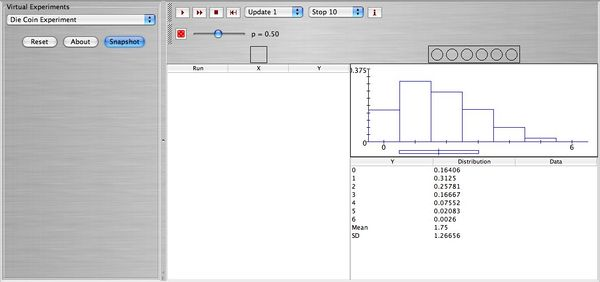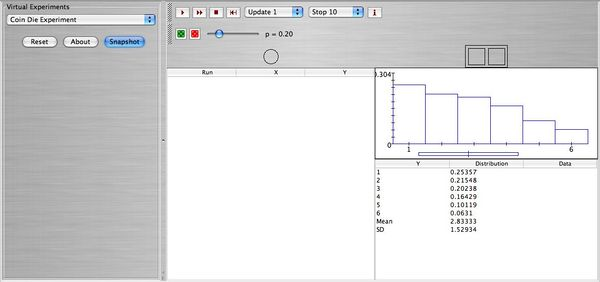# SOCR EduMaterials Activities JointDistributions

(diff) ← Older revision | Latest revision (diff) | Newer revision → (diff)

## This is an activity to explore the joint distributions of X and Y through two simple examples.

• Description: You can access the applets for the following experiments at SOCR Experiments
• Exercise 1: Die coin experiment:

A die is rolled and the number observed \( X \) is recorded. Then a coin is tossed number of times equal to the value of \(X\). For example if \(X=2\) then the coin is tossed twice, etc. Let \(Y\) be the number of heads observed. Note: Assume that the die and the coin are fair.

• 1. Construct the joint probability distribution of \(X\) and \(Y\).
• 2. Find the conditional expected value of \(Y\) given \(X=5\).
• 3. Find the conditional variance of \(Y\) given \(X=5\).
• 4. Find the expected value of \(Y\).
• 5. Find the standard deviation of \(Y\).
• 6. Graph the probability distribution of \(Y\).
• 7. Use SOCR to graph and print the empirical distribution of \(Y\) when the experiment is performed
• a. \(n=1000\) times.
• b. \(n=10000\) times.
• 8. Compare the theoretical mean and standard deviation of \(Y\) (parts (4) and (5)) with the empirical mean and standard deviation found in part (8).

Below you can see a snapshot of the theoretical distribution of \( Y \).• Exercise 2: Coin Die experiment:

A coin is tossed and if heads is observed then a red die is rolled. If tails is observed then a green die is rolled. You can choose the distribution of each die as well as the probability of heads. Choose for the red die the 3-4 flat distribution and for the green die the skewed right distribution. Finally using the scroll button choose \$p=0.2\$ as the probability of heads. Let \(X\) be the score of the coin (1 for heads, 0 for tails), and let \(Y\) be the score of the die (1,2,3,4,5,6).

• 1. Construct the joint probability distribution of \(X, Y\).
• 2. Find the marginal probability distribution of \(Y\) and verify that it is the same with the one given in the applet.
• 3. Compute \(E(Y)\).
• 4. Compute \(E(Y)\) using expectation by conditioning \(E[E(Y|X)]\).
• 5. Run the experiment 1000 times take a snapshot and comment on the results.

Below you can see a snapshot of the theoretical distribution of \( Y \).